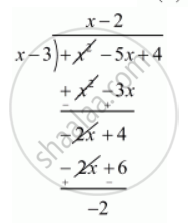Advertisement Remove all ads

# What Should Be Added to the Polynomial X2 − 5x + 4, So that 3 is the Zero of the Resulting Polynomial? - Mathematics

MCQ

What should be added to the polynomial x2 − 5x + 4, so that 3 is the zero of the resulting polynomial?

#### Options

• 1

• 2

• 4

• 5

Advertisement Remove all ads

#### Solution

If x = alpha, is a zero of a polynomial then x -alpha is a factor of  f(x)

Since 3 is the zero of the polynomial , f(x) = x2 − 5x + 4,

Therefore x - 3is a factor of  f(x)

Now, we dividef(x) = x^2 - 5x + 4  by  (x - 3) we getTherefore we should add 2 to the given polynomial

Hence, the correct choice is (b).

Is there an error in this question or solution?
Advertisement Remove all ads

#### APPEARS IN

RD Sharma Class 10 Maths
Chapter 2 Polynomials
Q 24 | Page 64
Advertisement Remove all ads

#### Video TutorialsVIEW ALL 

Advertisement Remove all ads
Share
Notifications

View all notifications

Forgot password?
Course